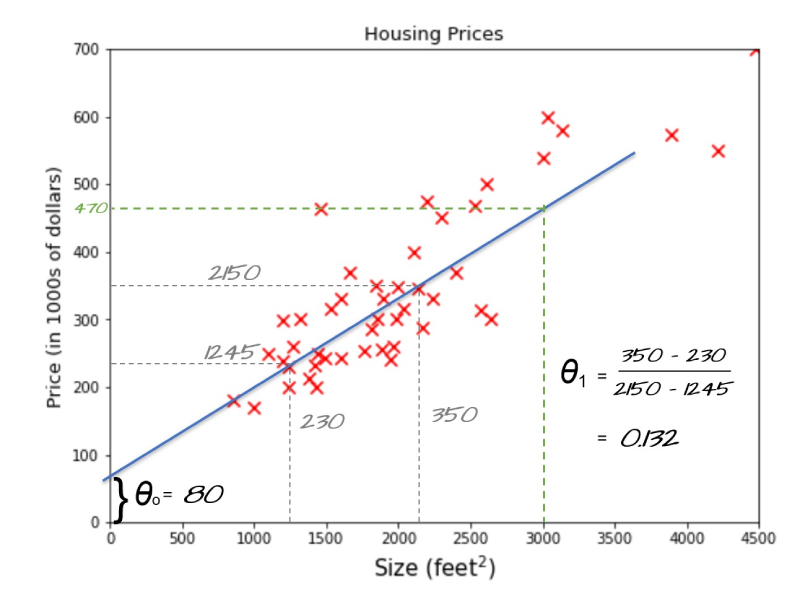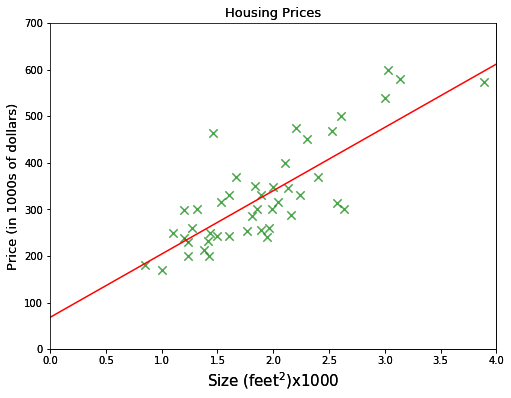• +91-9872993883
• +91-8283824812
• info@ris-ai.com

# House Price Prediction using Linear Regression

Project Objective: The main objective of this model is to predict the price of house on the basis of house size with the help of Linear Regression. It can be usefull for those who are in the bussiness of house retail bussiness so that they know the estimated value of the house for there further trades and in bussiness profits.

Firstly, we import necessary library(numpy, matplotlib and pandas) for this model.

In :
import pandas as pd
import matplotlib.pyplot as plt
import numpy as np


We read CSV file in which contains data for predicting house price. Names of the field is house size and house price .head() function will show the 5 dataset from the data.

In :
housing_data =  pd.read_csv('house-price-prediction-using-linear-regression.txt', usecols = [0,2],names=['size','price'])
#(we got this housing data from coursera-machine-learning-course-ex1)

Out:
size price
0 2104 399900
1 1600 329900
2 2400 369000
3 1416 232000
4 3000 539900

Here, we use Gradient Descent to minimize the cost function. The cost function only works when it knows the parameters’ values, In the above sample example we manually choose the parameters’ value each time but during the algorithmic calculation once the parameters’ values are randomly initialized it’s the gradient descent who have to decide what params value to choose in the next iteration in order to minimize the error, it’s the gradient descent who decide by how much to increase or decrease the params values

In :
def gradient_descent(alpha, n_iters):
X = housing_data['size'].values/1000
y = housing_data['price'].values/1000
m=len(X)
theta0 = 0
theta1 = 0
for _ in range(n_iters):
d_theta0 = []
d_theta1 = []
for i in range(m):
d_theta0.append((theta0+theta1*X[i])-y[i])
d_theta1.append(((theta0+theta1*X[i])-y[i])*X[i])
theta0 = theta0-alpha*(1/m)*sum(d_theta0)
theta1 = theta1-alpha*(1/m)*sum(d_theta1)

return theta0,theta1
print('intercept_term(theta0):',theta0,'\n','bias_term(theta1):',theta1)

intercept_term(theta0): 68.12351315549327
bias_term(theta1): 135.9217432231492


A company name ABC provides you a data on the houses’ size and its price. The company requires providing them a machine learning model that can predict houses’ prices for any given size. Let’s say what would be the best-estimated price for area 3000 feet square? If you are thinking to fit a line somewhere between the dataset and draw a verticle line from 3000 on the x-axis until it touches the line and then the corresponding value on the y-axis i.e 470 would be the answer, then you are on right track, it is represented by the green dotted line in the figure below.The algorithm working principle is the same for any number of parameters, it’s just that the more the parameters more the direction of the slope. Now the algorithm needs to look for both directions in order to minimize the cost function.

In :
hypothesis = theta0+np.dot(theta1,[0,4])
x=[0,4]
%matplotlib inline
plt.figure(figsize=(8,6))
plt.scatter(housing_data['size']/1000,housing_data['price']/1000, c='g',marker='x',s=70, alpha=0.7);
plt.plot(x,hypothesis, c='r')
plt.xlabel('Size (feet$^2$)x1000',size=15)
plt.ylabel('Price (in 1000s of dollars)', size=13)
plt.title('Housing Prices',size=13)
plt.plot()
plt.xlim(0,4)
plt.ylim(0,700)

Out:
(0, 700)Here, we predict the value of house by input the size value i.e. X here it is 3.

In :
def predict(X):
return theta0 + theta1*X
predict(3)

Out:
475.8887428249409